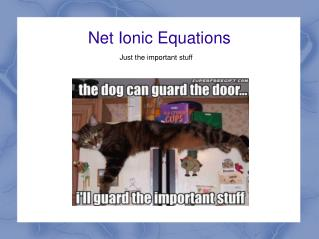DownloadDownload PresentationNet Ionic Equations

# Net Ionic Equations

Télécharger la présentation## Net Ionic Equations

- - - - - - - - - - - - - - - - - - - - - - - - - - - E N D - - - - - - - - - - - - - - - - - - - - - - - - - - -
##### Presentation Transcript

1. Net Ionic Equations Just the important stuff

2. Precipitation Reactions This concept applies to any reaction with an aqueous reactant, but it's easiest to see in precipitation reactions. Let's start with an example one: NaCl (aq) + AgNO3 (aq) → AgCl (s) + NaNO3 (aq)

3. Precipitation Reactions When we start, we have two beakers that look something like this (waters grayed out for clarity): Na+Cl-Ag+NO3-

4. Precipitation Reactions Afterwards, it looks something like this: AgCl + Na+ + NO3-

5. Precipitation Reactions Something clearly happened to the silver(I) and chloride—they combined to make a solid. But did the sodium and the nitrate really change? They started out dissolved, and were still dissolved after the reaction.

6. Spectator Ions NaCl (aq) + AgNO3 (aq) → AgCl (s) + NaNO3 (aq) In our example, the sodium and nitrate are spectator ions—ones which do not actually react; they're just there to balance out the charges, but don't do anything.

7. Net Ionic Equation NaCl (aq) + AgNO3 (aq) → AgCl (s) + NaNO3 (aq) The net ionic equation is one that simply takes out all the spectator ions, and shows only the ions that react: Ag+ + Cl-→ AgCl This is the net ionic equation for our reaction (note: the order doesn't matter, and anything written with a charge is assumed to be aqueous; you don't have to write the “(aq)”)

8. So Why Do This? 1. It's more simple—it shows only the things doing the actual reacting.

9. So Why Do This? 1. It's more simple—it shows only the things doing the actual reacting. 2. It lets us see when two seemingly-different reactions are actually the same. Consider our example, and a new one: NaCl (aq) + AgNO3 (aq) → AgCl (s) + NaNO3 (aq) 2KCl (aq) + Ag2SO4 (aq) → 2AgCl (s) + K2SO4 (aq) These look like completely different reactions if we write them out this way, but the net ionic equation for each of them is:

10. So Why Do This? 1. It's more simple—it shows only the things doing the actual reacting. 2. It lets us see when two seemingly-different reactions are actually the same. Consider our example, and a new one: NaCl (aq) + AgNO3 (aq) → AgCl (s) + NaNO3 (aq) 2KCl (aq) + Ag2SO4 (aq) → 2AgCl (s) + K2SO4 (aq) These look like completely different reactions if we write them out this way, but the net ionic equation for each of them is: Ag+ + Cl-→ AgCl These are the same reaction; the stuff doing the actual reacting is the same. The only difference is the completely irrelevant stuff we've paired them with. (Note: you balance net ionic equations on their own. The second one doesn't need twos just because the full equations had them as coefficients)

11. Last Reason 3. Sometimes, your “reaction” isn't really a reaction at all! NaCl (aq) + KNO3 (aq) → NaNO3 (aq) + KCl (aq) If we look at the before pictures again: Na+Cl-K+NO3-

12. Last Reason 3. Sometimes, your “reaction” isn't really a reaction at all! After mixing, nothing has really changed. We still just have all the same things dissolved; they're just dissolved in the same water now. Na+Cl-K+NO3-

13. Last Reason 3. Sometimes, your “reaction” isn't really a reaction at all! NaCl (aq) + KNO3 (aq) → NaNO3 (aq) + KCl (aq) Our net ionic equation, after we take out all the things that don't change, is: → Becausethis is not really a reaction in the first place. It's just mixing two things and writing them in a different way.

14. Some Examples Molecular: MgCl2 (aq) + 2KOH (aq) → Mg(OH)2 (s) + 2KCl (aq) Net ionic: Molecular: Na2CO3 (aq) + ZnBr2 (aq) → 2NaBr (aq) + ZnCO3 (s) Net ionic: Molecular: Ag2CO3 (s) + 2HCl (aq) → AgCl (s) + H2O (l) + CO2 (g) Net ionic: (This one is a very unusual case)

15. Some Examples Molecular: MgCl2 (aq) + 2KOH (aq) → Mg(OH)2 (s) + 2KCl (aq) Net ionic: Mg2+ + 2OH-→ Mg(OH)2 Molecular: Na2CO3 (aq) + ZnBr2 (aq) → 2NaBr (aq) + ZnCO3 (s) Net ionic: Zn2+ + CO32-→ ZnCO3 Molecular: Ag2CO3 (s) + 2HCl (aq) → AgCl (s) + H2O (l) + CO2 (g) Net ionic: Ag2CO3 + 2H+ + 2Cl-→ 2AgCl + H2O + CO2 (This one is a very unusual case)

16. Summary 1. Ions that start dissolved, and end dissolved, do not actually react 2. They are referred to as “spectator ions” 3. The net ionic equation shows only the ions that react, and no spectator ions. 4. If two reactions have the same net ionic equation, they are the same reaction 5. If there is no net ionic equation, it's not really a reaction at all.